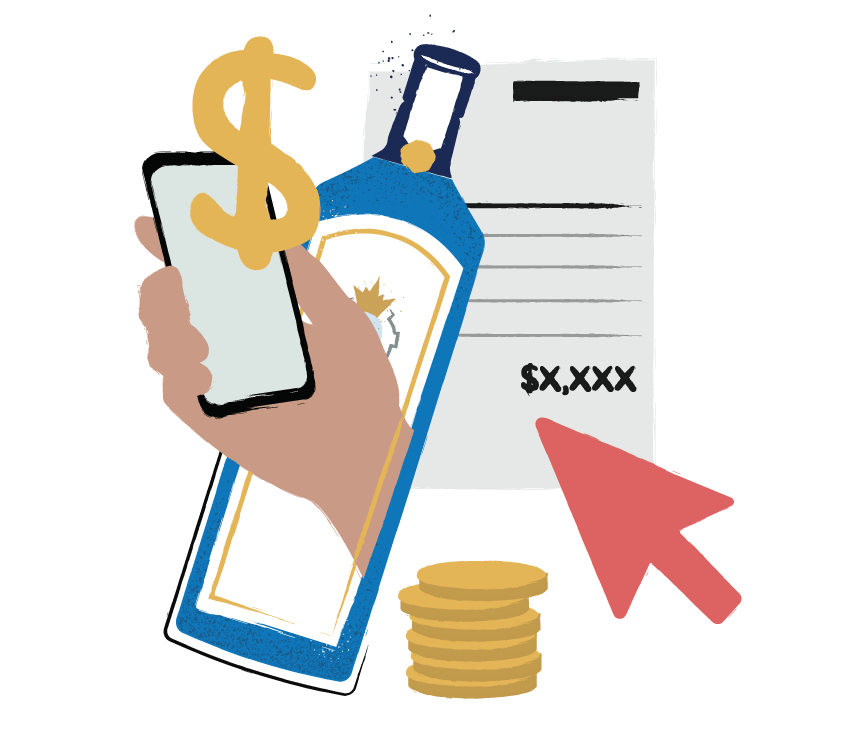## Liquor Cost Formula Cheat Sheet

This PDF cheat sheet provides useful formulas to help you calculate and better understand your bar performance.## Free Liquor Cost Formula Cheat Sheet

Understanding your alcoholic beverage numbers is critical to success, but there are a lot of formulas and metrics to remember. That's where our cheat sheet comes in. A table of the most useful formulas you'll need to calculate liquor cost metrics and analyze your restaurant or bar's performance.

The PDF Cheat Sheet includes the following formulas:

• Cost of Goods Sold & Usage
• Pour Cost
• Gross Margin
• Variance & Shrinkage
• Inventory Turnover
• Inventory Turn Days